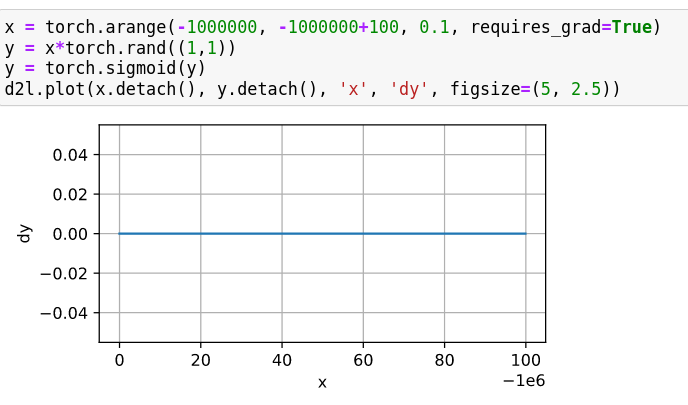# Multilayer Perceptrons

1. the derivative of the tanh:
dtanh(x)/dx=1−tanh^(2)=
{2exp(−2x)-[exp(−2x)]^2}/(1+exp(−2x))^2.
https://www.math24.net/derivatives-hyperbolic-functions/
the derivative of the pReLU(x):
dpReLU(x)/dx = 1 (if x > 0);α (if x < 0);doesn’t exist (if x = 0)
2. h= ReLU(x) = max(x, 0)
y = ReLU(h) = max(h, 0) = max (x, 0) =ReLU(x)
h= pReLU(x)=max(0,x)+αmin(0,x).
y = pReLU(h) =max(0,h)+αmin(0,h)
One linear functions add,minus other linear function still is linear function.
3. [1−exp(−2x)]/[1+exp(−2x)]+1 = 2/[1+exp(−2x)] = 2 * 1/[1+exp(−2x)] = 2 sigmoid(2x).
4. d/2 dimensions will cause linearly dependent?
5. overfit?
1 Like

The section for plotting the gradient relu function.

y.backward(torch.ones_like(x), retain_graph=True)

Should there be a

y.backward(torch.ones_like(x), retain_graph=True)

Else if we run the notebook twice the gradient will keep on adding

Sure @sushmit86 that’s a genuine concern and would be a good idea but we don’t want to add complexity to the book content.

If you feel the need to run the cells twice you can add the extra line to zero out the gradsThanks so much for the reply

# Question 2:

I think it should be this:

• H = Relu(XW^(1) + b^(2))
• y = HW^(2) + b^(2)

More detail in page 131.
I think it is more easy to think like this:
Relu(x) constructs a continuous piecewise linear function for every x\in R. So, it do not depend on whatever x is providing that x is continuous in R. So, Relu(Relu(x)*W+b) for example is also constructs a continuous piecewise linear function.

# Question 4:

I think the most different between an MLP apply nonlinearity and MLP not apply nonlinearity is the time and complexity. In fact, MLPs applying nonlinearity such as Sigmoid and tanh are very expensive to calculate and find the derivative for gradient descent. So, we need something faster and Relu is a good choice to address these problem (6.x sigmoid).

2 Likes

Ans for question 4

I think if we apply different non linearity for different mini batches , as the activation function changes the first thing is the range of the output will vary which we affect the final output

### Exeercises

1. Compute the derivative of the pReLU activation function.
• made a way to describe the function but the torch autograd is not able to work
alpha = 0.1

y = find_max(X) + alpha * find_min(X)
2. Show that an MLP using only ReLU (or pReLU) constructs a continuous piecewise linear

function.

• I guess we need to construct a multi layer perceptron here. dontknow.
1. Show that tanh(x) + 1 = 2 sigmoid(2x).
• through plottinga graph we can show.

1. Assume that we have a nonlinearity that applies to one minibatch at a time. What kinds of

problems do you expect this to cause?

• maybe this would create problems like each min batch would be squished(scaled) differently.

Hi, @goldpiggy
I have a question regarding eq (4.1.1), consequently the rest.
If X is a n *d matrix and W^(1) is a d *h matrix, then both H and XW^(1) is a n*h matrix. However, b^(1) is a 1*h row vector? The dimension of this equation is not consistent. Should it be b^(1) is a n*h matrix?
Similarly for b^(2)

Does deep learning framwork (like PyTorch, Tensorflow, …) use some tricks when calculating the derivative like sigmoid, tanh (exponential family) ?D sigmoid(x) = sigmoid(x) * (1 - sigmoid(x)) can efficiently decrease computational work even if they use computational graph with backpropagation.

However, this also reduces the portability of the framework.

Here are my opinions for the exercises:
I don’t know the answer for ex.6ex.1
The derivative for any x in a multi-layer perceptron nuralnetwork is always a fixed value, that is this kind of nn is always linear.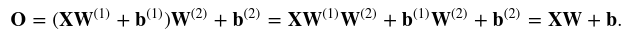And if the weight of second layer has less dimensions than the first, the dimension of W model is reduced, which means a reduction of expressive capacity.

ex.2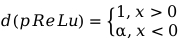ex.3

ex.4

x_1 = torch.arange(-8.0, 8.0, 0.1)
w_1 = torch.rand(1, 1)
b_1 = torch.rand(1, 1)
y_1 = x_1*w_1+b_1
x_2 = torch.relu(y_1)
w_2 = torch.rand(1, 1)
b_2 = torch.rand(1, 1)
y_2 = x_2*w_2+b_2
d2l.plot(x.detach(), y_2.detach(), 'x', 'nn_withReLu(x)')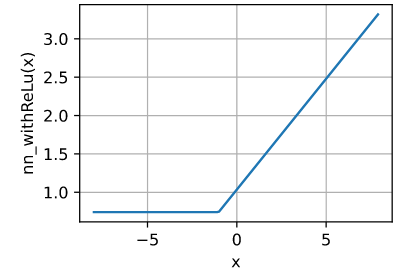x_1 = torch.arange(-8.0, 8.0, 0.1)
w_1 = torch.rand(1, 1)
b_1 = torch.rand(1, 1)
y_1 = x_1*w_1+b_1
x_2 = torch.prelu(y_1,torch.tensor(0.5))
w_2 = torch.rand(1, 1)
b_2 = torch.rand(1, 1)
y_2 = x_2*w_2+b_2
d2l.plot(x.detach(), y_2.detach(), 'x', 'nn_withpReLu(x)')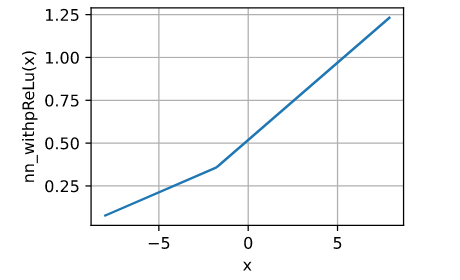ex.5
A.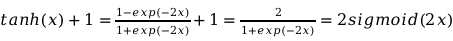B. As shown in A, tanh(x) is kinda like adding a affine layer just behind the activation stage with sigmoid , and considering the conclusion in ex1, this kind of affine layer can merge with the affine layer behind to make it identical to a nn using sigmoid, vice versa.

ex.6
??

ex.7